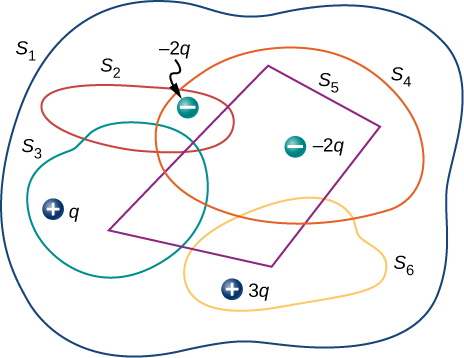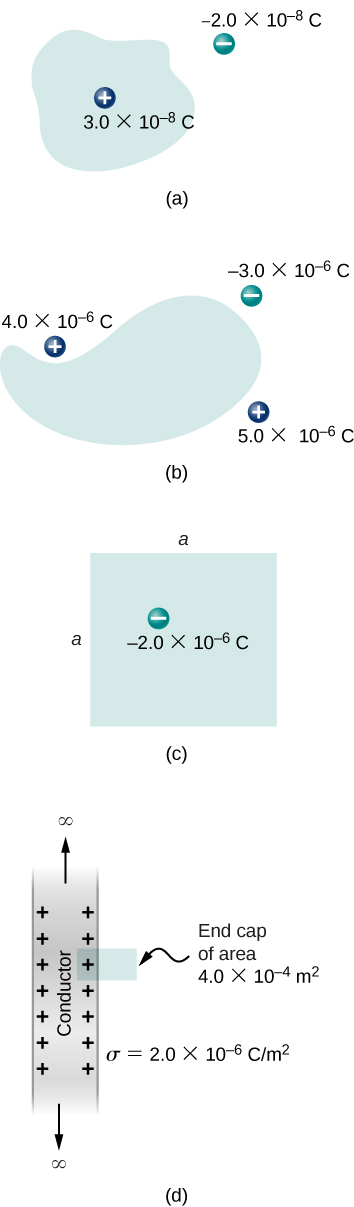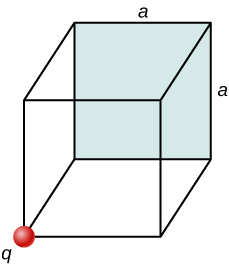6.2 Explaining gauss’s law  (Page 3/4)

 Page 3 / 4

Gauss’s law

The flux $\text{Φ}$ of the electric field $\stackrel{\to }{\text{E}}$ through any closed surface S (a Gaussian surface) is equal to the net charge enclosed $\left({q}_{\text{enc}}\right)$ divided by the permittivity of free space $\left({\epsilon }_{0}\right):$

$\text{Φ}={\oint }_{S}\stackrel{\to }{\text{E}}·\stackrel{^}{\text{n}}\phantom{\rule{0.2em}{0ex}}dA=\frac{{q}_{\text{enc}}}{{\epsilon }_{0}}.$

To use Gauss’s law effectively, you must have a clear understanding of what each term in the equation represents. The field $\stackrel{\to }{\text{E}}$ is the total electric field at every point on the Gaussian surface. This total field includes contributions from charges both inside and outside the Gaussian surface. However, ${q}_{\text{enc}}$ is just the charge inside the Gaussian surface. Finally, the Gaussian surface is any closed surface in space. That surface can coincide with the actual surface of a conductor, or it can be an imaginary geometric surface. The only requirement imposed on a Gaussian surface is that it be closed ( [link] ).A Klein bottle partially filled with a liquid. Could the Klein bottle be used as a Gaussian surface?

Electric flux through gaussian surfaces

Calculate the electric flux through each Gaussian surface shown in [link] .

Strategy

From Gauss’s law, the flux through each surface is given by ${q}_{\text{enc}}\text{/}{\epsilon }_{0},$ where ${q}_{\text{enc}}$ is the charge enclosed by that surface.

Solution

For the surfaces and charges shown, we find

1. $\text{Φ}=\frac{2.0\phantom{\rule{0.2em}{0ex}}\mu \text{C}}{{\epsilon }_{0}}=2.3\phantom{\rule{0.2em}{0ex}}×\phantom{\rule{0.2em}{0ex}}{10}^{5}\phantom{\rule{0.2em}{0ex}}\text{N}·{\text{m}}^{2}\text{/C}\text{.}$
2. $\text{Φ}=\frac{-2.0\phantom{\rule{0.2em}{0ex}}\mu \text{C}}{{\epsilon }_{0}}=-2.3\phantom{\rule{0.2em}{0ex}}×\phantom{\rule{0.2em}{0ex}}{10}^{5}\phantom{\rule{0.2em}{0ex}}\text{N}·{\text{m}}^{2}\text{/C}\text{.}$
3. $\text{Φ}=\frac{2.0\phantom{\rule{0.2em}{0ex}}\mu \text{C}}{{\epsilon }_{0}}=2.3\phantom{\rule{0.2em}{0ex}}×\phantom{\rule{0.2em}{0ex}}{10}^{5}\phantom{\rule{0.2em}{0ex}}\text{N}·{\text{m}}^{2}\text{/C}\text{.}$
4. $\text{Φ}=\frac{-4.0\phantom{\rule{0.2em}{0ex}}\mu \text{C}+6.0\phantom{\rule{0.2em}{0ex}}\mu \text{C}-1.0\phantom{\rule{0.2em}{0ex}}\mu \text{C}}{{\epsilon }_{0}}=1.1\phantom{\rule{0.2em}{0ex}}×\phantom{\rule{0.2em}{0ex}}{10}^{5}\phantom{\rule{0.2em}{0ex}}\text{N}·{\text{m}}^{2}\text{/C}\text{.}$
5. $\text{Φ}=\frac{4.0\phantom{\rule{0.2em}{0ex}}\mu \text{C}+6.0\phantom{\rule{0.2em}{0ex}}\mu \text{C}-10.0\phantom{\rule{0.2em}{0ex}}\mu \text{C}}{{\epsilon }_{0}}=0.$

Significance

In the special case of a closed surface, the flux calculations become a sum of charges. In the next section, this will allow us to work with more complex systems.

Check Your Understanding Calculate the electric flux through the closed cubical surface for each charge distribution shown in [link] .

a. $3.4\phantom{\rule{0.2em}{0ex}}×\phantom{\rule{0.2em}{0ex}}{10}^{5}\phantom{\rule{0.2em}{0ex}}\text{N}·{\text{m}}^{2}\text{/C;}$ b. $-3.4\phantom{\rule{0.2em}{0ex}}×\phantom{\rule{0.2em}{0ex}}{10}^{5}\phantom{\rule{0.2em}{0ex}}\text{N}·{\text{m}}^{2}\text{/C;}$ c. $3.4\phantom{\rule{0.2em}{0ex}}×\phantom{\rule{0.2em}{0ex}}{10}^{5}\phantom{\rule{0.2em}{0ex}}\text{N}·{\text{m}}^{2}\text{/C;}$ d. 0

Use this simulation to adjust the magnitude of the charge and the radius of the Gaussian surface around it. See how this affects the total flux and the magnitude of the electric field at the Gaussian surface.

Summary

• Gauss’s law relates the electric flux through a closed surface to the net charge within that surface,
$\text{Φ}={\oint }_{S}\stackrel{\to }{\text{E}}·\stackrel{^}{\text{n}}\phantom{\rule{0.2em}{0ex}}dA=\frac{{q}_{\text{enc}}}{{\epsilon }_{0}},$

where ${q}_{\text{enc}}$ is the total charge inside the Gaussian surface S .
• All surfaces that include the same amount of charge have the same number of field lines crossing it, regardless of the shape or size of the surface, as long as the surfaces enclose the same amount of charge.

Conceptual questions

Two concentric spherical surfaces enclose a point charge q . The radius of the outer sphere is twice that of the inner one. Compare the electric fluxes crossing the two surfaces.

Since the electric field vector has a $\frac{1}{{r}^{2}}$ dependence, the fluxes are the same since $A=4\pi {r}^{2}$ .

Compare the electric flux through the surface of a cube of side length a that has a charge q at its center to the flux through a spherical surface of radius a with a charge q at its center.

(a) If the electric flux through a closed surface is zero, is the electric field necessarily zero at all points on the surface? (b) What is the net charge inside the surface?

a. no; b. zero

Discuss how Gauss’s law would be affected if the electric field of a point charge did not vary as $1\text{/}{r}^{2}.$

Discuss the similarities and differences between the gravitational field of a point mass m and the electric field of a point charge q .

Both fields vary as $\frac{1}{{r}^{2}}$ . Because the gravitational constant is so much smaller than $\frac{1}{4\pi {\epsilon }_{0}}$ , the gravitational field is orders of magnitude weaker than the electric field.

Discuss whether Gauss’s law can be applied to other forces, and if so, which ones.

Is the term $\stackrel{\to }{E}$ in Gauss’s law the electric field produced by just the charge inside the Gaussian surface?

No, it is produced by all charges both inside and outside the Gaussian surface.

Reformulate Gauss’s law by choosing the unit normal of the Gaussian surface to be the one directed inward.

Problems

Determine the electric flux through each surface whose cross-section is shown below.Find the electric flux through the closed surface whose cross-sections are shown below.a. $\text{Φ}=3.39\phantom{\rule{0.2em}{0ex}}×\phantom{\rule{0.2em}{0ex}}{10}^{3}\phantom{\rule{0.2em}{0ex}}\text{N}·{\text{m}}^{2}\text{/}\text{C}$ ; b. $\text{Φ}=0$ ;
c. $\text{Φ}=-2.25\phantom{\rule{0.2em}{0ex}}×\phantom{\rule{0.2em}{0ex}}{10}^{5}\phantom{\rule{0.2em}{0ex}}\text{N}·{\text{m}}^{2}\text{/}\text{C}$ ;
d. $\text{Φ}=90.4\phantom{\rule{0.2em}{0ex}}\text{N}·{\text{m}}^{2}\text{/}\text{C}$

A point charge q is located at the center of a cube whose sides are of length a . If there are no other charges in this system, what is the electric flux through one face of the cube?

A point charge of $10\phantom{\rule{0.2em}{0ex}}\mu \text{C}$ is at an unspecified location inside a cube of side 2 cm. Find the net electric flux though the surfaces of the cube.

$\text{Φ}=1.13\phantom{\rule{0.2em}{0ex}}×\phantom{\rule{0.2em}{0ex}}{10}^{6}\phantom{\rule{0.2em}{0ex}}\text{N}·{\text{m}}^{2}\text{/C}$

A net flux of $1.0\phantom{\rule{0.2em}{0ex}}×\phantom{\rule{0.2em}{0ex}}{10}^{4}\phantom{\rule{0.2em}{0ex}}\text{N}·{\text{m}}^{2}\text{/C}$ passes inward through the surface of a sphere of radius 5 cm. (a) How much charge is inside the sphere? (b) How precisely can we determine the location of the charge from this information?

A charge q is placed at one of the corners of a cube of side a , as shown below. Find the magnitude of the electric flux through the shaded face due to q . Assume $q>0$ .Make a cube with q at the center, using the cube of side a . This would take four cubes of side a to make one side of the large cube. The shaded side of the small cube would be 1/24th of the total area of the large cube; therefore, the flux through the shaded area would be
$\text{Φ}=\frac{1}{24}\phantom{\rule{0.2em}{0ex}}\frac{q}{{\epsilon }_{0}}$ .

The electric flux through a cubical box 8.0 cm on a side is $1.2\phantom{\rule{0.2em}{0ex}}×\phantom{\rule{0.2em}{0ex}}{10}^{3}\phantom{\rule{0.2em}{0ex}}\text{N}·{\text{m}}^{2}\text{/C}\text{.}$ What is the total charge enclosed by the box?

The electric flux through a spherical surface is $4.0\phantom{\rule{0.2em}{0ex}}×\phantom{\rule{0.2em}{0ex}}{10}^{4}\phantom{\rule{0.2em}{0ex}}\text{N}·{\text{m}}^{2}\text{/C}\text{.}$ What is the net charge enclosed by the surface?

$q=3.54\phantom{\rule{0.2em}{0ex}}×\phantom{\rule{0.2em}{0ex}}{10}^{-7}\phantom{\rule{0.2em}{0ex}}\text{C}$

A cube whose sides are of length d is placed in a uniform electric field of magnitude $E=4.0\phantom{\rule{0.2em}{0ex}}×\phantom{\rule{0.2em}{0ex}}{10}^{3}\phantom{\rule{0.2em}{0ex}}\text{N/C}$ so that the field is perpendicular to two opposite faces of the cube. What is the net flux through the cube?

Repeat the previous problem, assuming that the electric field is directed along a body diagonal of the cube.

zero, also because flux in equals flux out

A total charge $5.0\phantom{\rule{0.2em}{0ex}}×\phantom{\rule{0.2em}{0ex}}{10}^{-6}\phantom{\rule{0.2em}{0ex}}\text{C}$ is distributed uniformly throughout a cubical volume whose edges are 8.0 cm long. (a) What is the charge density in the cube? (b) What is the electric flux through a cube with 12.0-cm edges that is concentric with the charge distribution? (c) Do the same calculation for cubes whose edges are 10.0 cm long and 5.0 cm long. (d) What is the electric flux through a spherical surface of radius 3.0 cm that is also concentric with the charge distribution?

What is differential form of Gauss's law?
help me out on this question the permittivity of diamond is 1.46*10^-10.( a)what is the dielectric of diamond (b) what its susceptibility
a body is projected vertically upward of 30kmp/h how long will it take to reach a point 0.5km bellow e point of projection
i have to say. who cares. lol. why know that t all
Jeff
is this just a chat app about the openstax book?
kya ye b.sc ka hai agar haa to konsa part
what is charge quantization
it means that the total charge of a body will always be the integral multiples of basic unit charge ( e ) q = ne n : no of electrons or protons e : basic unit charge 1e = 1.602×10^-19
Riya
is the time quantized ? how ?
Mehmet
What do you meanby the statement,"Is the time quantized"
Mayowa
Can you give an explanation.
Mayowa
there are some comment on the time -quantized..
Mehmet
time is integer of the planck time, discrete..
Mehmet
planck time is travel in planck lenght of light..
Mehmet
it's says that charges does not occur in continuous form rather they are integral multiple of the elementary charge of an electron.
Tamoghna
it is just like bohr's theory. Which was angular momentum of electron is intral multiple of h/2π
determine absolute zero
The properties of a system during a reversible constant pressure non-flow process at P= 1.6bar, changes from constant volume of 0.3m³/kg at 20°C to a volume of 0.55m³/kg at 260°C. its constant pressure process is 3.205KJ/kg°C Determine: 1. Heat added, Work done, Change in Internal Energy and Change in Enthalpy
U can easily calculate work done by 2.303log(v2/v1)
Abhishek
Amount of heat added through q=ncv^delta t
Abhishek
Change in internal energy through q=Q-w
Abhishek
please how do dey get 5/9 in the conversion of Celsius and Fahrenheit
what is copper loss
this is the energy dissipated(usually in the form of heat energy) in conductors such as wires and coils due to the flow of current against the resistance of the material used in winding the coil.
Henry
it is the work done in moving a charge to a point from infinity against electric field
what is the weight of the earth in space
As w=mg where m is mass and g is gravitational force... Now if we consider the earth is in gravitational pull of sun we have to use the value of "g" of sun, so we can find the weight of eaeth in sun with reference to sun...
Prince
g is not gravitacional forcé, is acceleration of gravity of earth and is assumed constante. the "sun g" can not be constant and you should use Newton gravity forcé. by the way its not the "weight" the physical quantity that matters, is the mass
Jorge
Yeah got it... Earth and moon have specific value of g... But in case of sun ☀ it is just a huge sphere of gas...
Prince
Thats why it can't have a constant value of g ....
Prince
not true. you must know Newton gravity Law . even a cloud of gas it has mass thats al matters. and the distsnce from the center of mass of the cloud and the center of the mass of the earth
Jorge
please why is the first law of thermodynamics greater than the second
every law is important, but first law is conservation of energy, this state is the basic in physics, in this case first law is more important than other laws..
Mehmet
First Law describes o energy is changed from one form to another but not destroyed, but that second Law talk about entropy of a system increasing gradually
Mayowa
first law describes not destroyer energy to changed the form, but second law describes the fluid drection that is entropy. in this case first law is more basic accorging to me...
Mehmet
define electric image.obtain expression for electric intensity at any point on earthed conducting infinite plane due to a point charge Q placed at a distance D from it.
explain the lack of symmetry in the field of the parallel capacitor
pls. explain the lack of symmetry in the field of the parallel capacitor
PhoebeBy Nick Swain